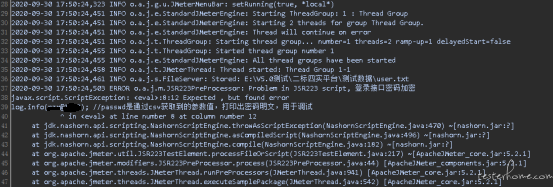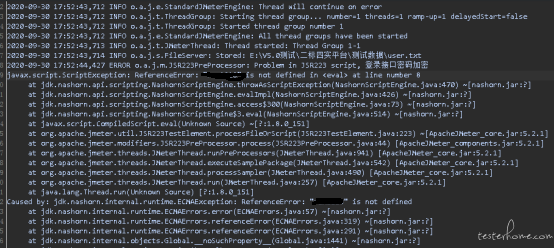# 性能测试工具 Jmeter 登录使用了 jsencrypt 加密密码的登录接口

``````const getEncrypt = (data) => {
let publickKey = '公钥字符串'
let crypt = new JSEncrypt()
crypt.setPublicKey(publickKey)
return crypt.encrypt(data)
}
``````

``````load("jsencrypt.js"); //加载js文件

var publicKey = "公钥字符串"; //找开发要的公钥秘钥
var crypt = new JSEncrypt();
crypt.setPublicKey(publicKey);//设置公钥秘钥
log.info("\${passwd}"); //passwd是通过csv获取到的参数值，打印出密码明文，用于调试
var passwdEncryption = crypt.encrypt("\${passwd}"); //对密码明文进行加密
log.info(passwdEncryption); //打印出秘密加密后样式
vars.put("passwdEncryption", passwdEncryption); //把加密后的放入全局变量中，然后在登录接口引用\${passwdEncryption}
``````

Jmeter 截图如下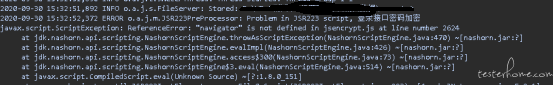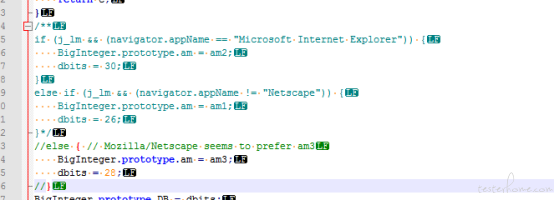navigator 和 window 变量相关代码都注释后，再执行 Jmeter 脚本。报了其他问题 JSEncrypt is not a function...。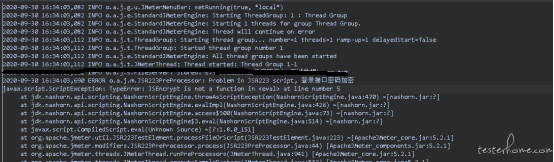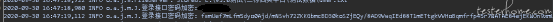``````var window = this; //定义window全局变量
var navigator = this; //定义navigator全局变量

var publicKey = "公钥字符串"; //找开发要的公钥秘钥
var crypt = new JSEncrypt();
crypt.setPublicKey(publicKey);//设置公钥秘钥
log.info("\${passwd}"); //passwd是通过csv获取到的参数值，打印出密码明文，用于调试
var passwdEncryption = crypt.encrypt("\${passwd}"); //对密码明文进行加密
log.info(passwdEncryption); //打印出密码加密后样式
vars.put("passwdEncryption", passwdEncryption); //把加密后的放入Jmeter全局变量中，然后在登录接口引用\${passwdEncryption}
``````